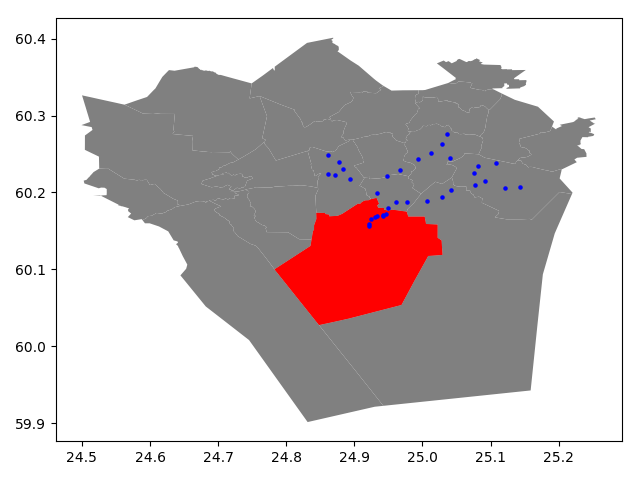# Count how many new points are captured when a polygon buffers by x miles

I have a polygon and a layer of many points. How many more points would be captured if I expanded the polygon by x meters? I want to end up with values for x = 1 mile, 5 miles, 10 miles, and maybe a few more. My instinct is to plug it into GIS, calculate a buffer, cut out the original polygon, and count point features, but it may not be feasible when I get more points: I will eventually be using a layer of tens of millions of points.

Is there a faster way to do this, maybe outside of a GIS?You can use `join attribute by nearest` to join the polygon (second input layer in GUI) to your points (first input layer). This adds the attributes of the polygon to the points (irrelevant for you, I know) and also the minimum distance between points and polygon. Then you may use `select by attribute` or a filter to get the points you want.

For such a high volume of points I recommend using optimized query processes. But it depends on what software you use.

If you use QGIS I recommend the process toolbox, specifically the Select by Location process, but it is very important that you previously generate the spatial index.

On the other hand, PostGIS, with the spatial indexes previously generated has a better performance.

Another aspect, that you should evaluate, analyzed by several authors, is that if the polygon is very large and covers the extent of the point cloud, in this case the spatial index is not very useful.

It would be advisable to cut your polygon into smaller parts, a grid, and calculate separately each one then accumulate the result.

Of course, this procedure is more practical using programming.

https://geoffboeing.com/2016/10/r-tree-spatial-index-python/#more-2183

Use this expression on the point layer to calculate the number of additional points covered by a buffer of `N` (here: `85`, change the value on line 16). Replace `poly` (line 9) with the name of your polygon layer:

``````sum(
if (
within (
\$geometry,
with_variable(
'polygon',
array_first (
overlay_nearest (
'poly',
\$geometry
)
),
difference (
buffer (
@polygon,
85  -- change this value for the buffer size
),
@polygon
)
)
),
1,
0
)
)
``````

Screenshot: initial polygon (blue) an buffer of 85 (yellow). The expression (here used to create a dynamic label for all points) shows the no. of additional points: those inside the yellow polygon: 17 in total: